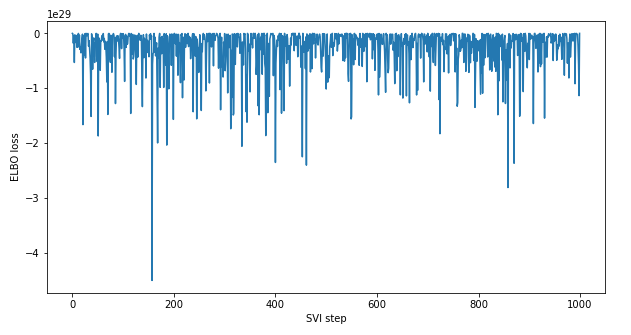# Advice on ELBO Loss Not Converging / Model Setup

The linear regression model below is currently not converging based on ELBO Loss. I’m using simulated data to test the model, and I’m not sure if the model is appropriately setup.

About the input data - each row in the data corresponds to one person, and the columns are attributes (i.e. x1, x2, x3, and y). Also, I’m attempting to use an associated network dataset (edges_df) to infer a latent impact score of the an individual’s connections, taking the mean impact score of a given individual’s connections as an input to the regression model.

About the outputs - in addition to estimating the regression coefficients, I would like to estimate the latent impact scores for each person. I’m looking for advice to improve the current model or an alternative approach that would yield valid and reliable results.

``````
def model(person_idx, edges_df, x1, x2, x3, y=None):
"""
person_idx: person index (i.e. unique identifier for each person)
edges_df: Pandas dataframe with "source" and "target" columns. The values in
the "target" column are lists of edges, e.g. [2, 17, 39]. Edge values
are based on person_idx.
x1 - x3: numeric input variables
y: numeric target
"""
# Coefficients
intercept = pyro.sample("intercept", dist.Normal(0., 1.0))
b_x1 = pyro.sample("b_x1", dist.Normal(0., 1.0))
b_x2 = pyro.sample("b_x2", dist.Normal(0., 1.0))
b_x3 = pyro.sample("b_x3", dist.Normal(0., 1.0))
b_emls = pyro.sample("b_emls", dist.Normal(0., 1.0))
sigma = pyro.sample("sigma", dist.HalfNormal(1.0))

# A unique latent score for each person
mu_ls = pyro.sample("mu_ls", dist.Normal(0.0, 1.0))
sigma_ls = pyro.sample("sigma_ls", dist.HalfNormal(1.0))
n_people = len(person_idx)
with pyro.plate("plate_ls", n_people):
latent_score = pyro.sample("latent_score", dist.Normal(mu_ls, sigma_ls))

# Mean latent score of edges
edges_mean_latent_impact_score = torch.empty_like(x1)
for p in person_idx:
# Returns list of edges for person p
p_edges = edges_df.loc[edges_df['source'] == p, 'target']
num_p_edges = len(p_edges)
if num_p_edges > 0:
p_edges_mean_latent_score = sum([latent_score[e] for e in p_edges]) / num_p_edges
edges_mean_latent_impact_score[p] = p_edges_mean_latent_score
else:
# Set value to 0 (i.e. neutral score) for those with no edges
edges_mean_latent_impact_score[p] = 0

mean = \
intercept +\
b_x1 * x1 +\
b_x2 * x2 +\
b_x3 * x3 +\
b_emls * edges_mean_latent_impact_score

with pyro.plate("data", len(x1)):
return pyro.sample("y_score", dist.Normal(mean, sigma), obs=y)

``````

Plate Model PNG:

Model Guide and SVI setup:

``````
mvn_guide = pyro.infer.autoguide.AutoMultivariateNormal(model)
svi = pyro.infer.SVI(
model,
mvn_guide,
pyro.infer.Trace_ELBO())

losses = []
for step in tqdm(range(1000)):
loss = svi.step(person_idx, edges_df, x1, x2, x3, y)
losses.append(loss)
if step % 100 == 0:
logging.info("Elbo loss: {}".format(loss))

# Visualize ELBO loss
plt.figure(figsize=(10, 5))
plt.plot(losses)
plt.xlabel("SVI step")
plt.ylabel("ELBO loss")

``````

ELBO Loss Plot:didn’t look at your model in much detail but here are some suggestions:

• read the tips and tricks tutorial. e.g. your learning rate is rather large. you should also see if you can get AutoDelta and AutoNormal to work before you try the `AutoMultivariateNormal`.
• demote your `sigma_*` latent variables to `pyro.param` statements. variational inference can work poorly when being bayesian about such parameters
1 Like

Great - thank you!

The suggestions you offered resolved the convergence issue. Thank you again!

Similar to the one above, do you know what might be causing the latent score to not be factoring into to the regression model as indicated by the following plate model png? More specifically, it seems like the `edges_mean_latent_impact_score` isn’t being included.

as far as i see `edges_mean_latent_impact_score` is not a `pyro.sample` statement so there’s no reason it should appear. it’s a deterministic intermediate computation. maybe it’ll appear if you mark it as such with `pyro.deterministic` i’m not sure. btw all the code in `model` gets executed during each elbo computation. so all data pre-processing should be removed from `model` and done once elsewhere. in particular you should get rid of all pandas computations and try to vectorize `for p in person_idx` if you want speed-ups.

1 Like

Thank you @martinjankowiak!